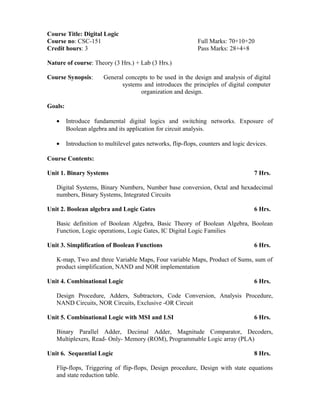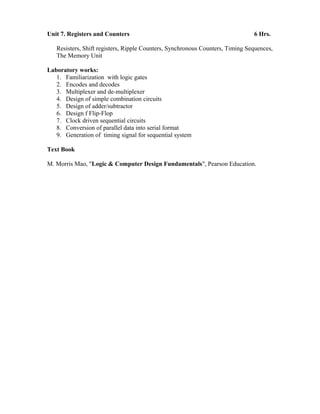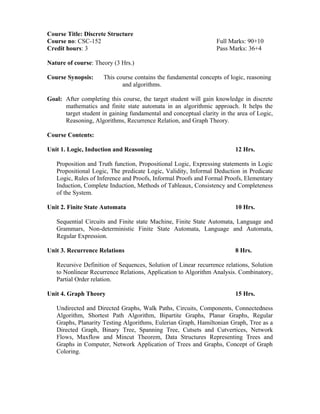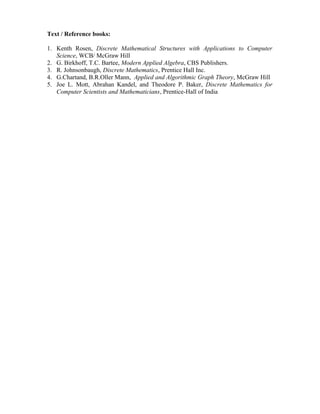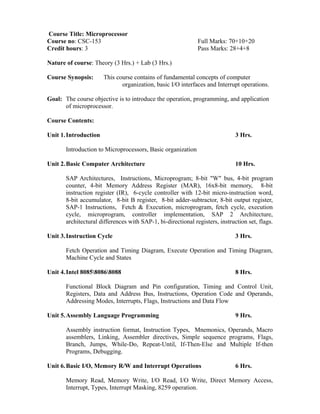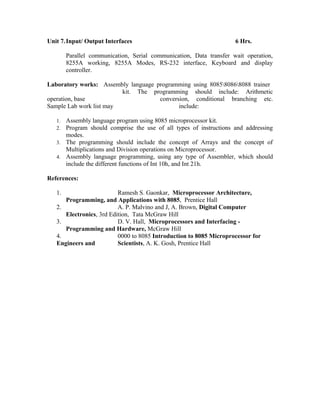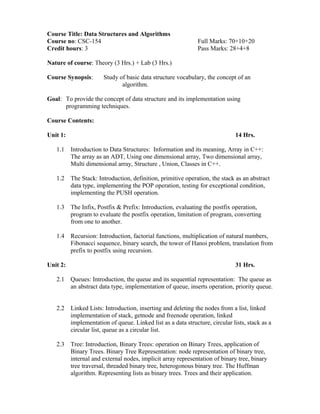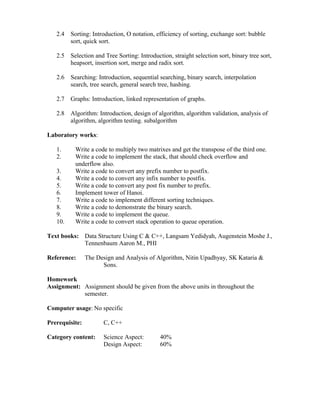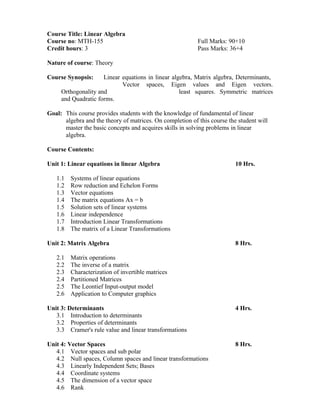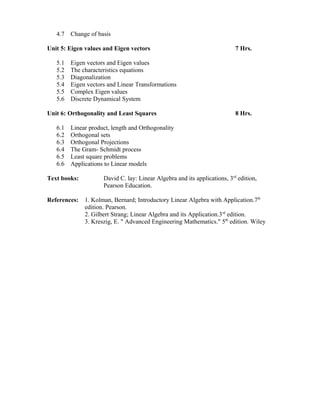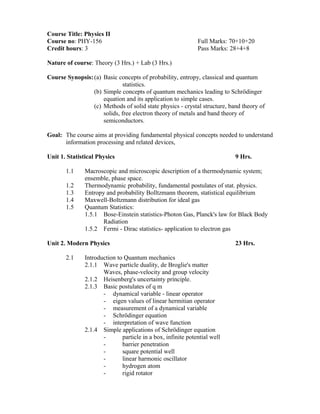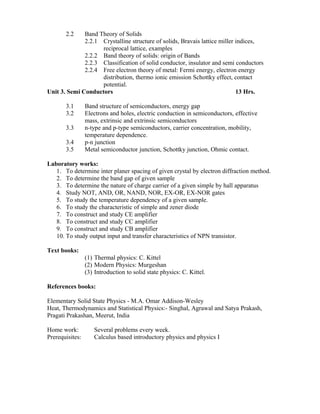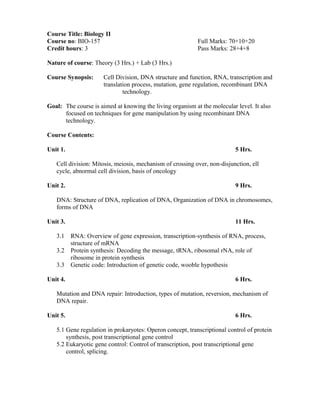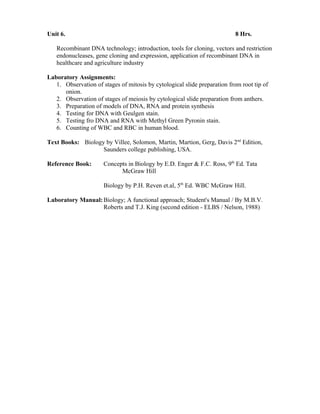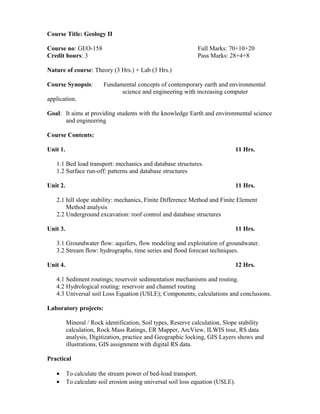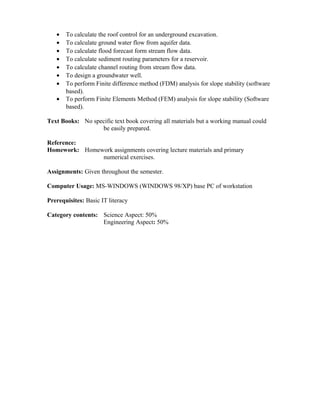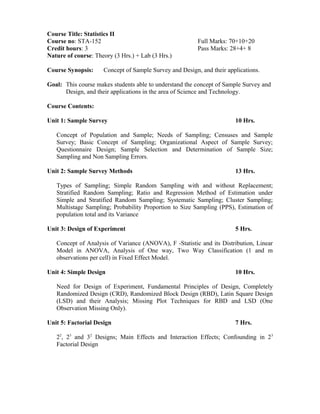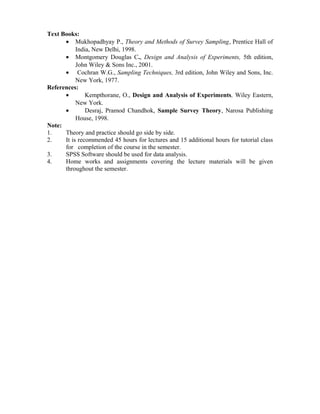Próximo SlideShare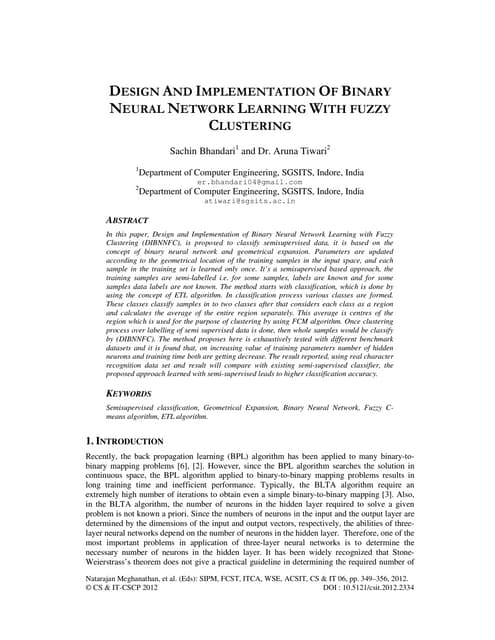DESIGN AND IMPLEMENTATION OF BINARY NEURAL NETWORK LEARNING WITH FUZZY CLUSTE...
Cargando en ... 3
1 de 18

### 2nd sem

1. Course Title: Digital Logic Course no: CSC-151 Full Marks: 70+10+20 Credit hours: 3 Pass Marks: 28+4+8 Nature of course: Theory (3 Hrs.) + Lab (3 Hrs.) Course Synopsis: General concepts to be used in the design and analysis of digital systems and introduces the principles of digital computer organization and design. Goals: • Introduce fundamental digital logics and switching networks. Exposure of Boolean algebra and its application for circuit analysis. • Introduction to multilevel gates networks, flip-flops, counters and logic devices. Course Contents: Unit 1. Binary Systems 7 Hrs. Digital Systems, Binary Numbers, Number base conversion, Octal and hexadecimal numbers, Binary Systems, Integrated Circuits Unit 2. Boolean algebra and Logic Gates 6 Hrs. Basic definition of Boolean Algebra, Basic Theory of Boolean Algebra, Boolean Function, Logic operations, Logic Gates, IC Digital Logic Families Unit 3. Simplification of Boolean Functions 6 Hrs. K-map, Two and three Variable Maps, Four variable Maps, Product of Sums, sum of product simplification, NAND and NOR implementation Unit 4. Combinational Logic 6 Hrs. Design Procedure, Adders, Subtractors, Code Conversion, Analysis Procedure, NAND Circuits, NOR Circuits, Exclusive -OR Circuit Unit 5. Combinational Logic with MSI and LSI 6 Hrs. Binary Parallel Adder, Decimal Adder, Magnitude Comparator, Decoders, Multiplexers, Read- Only- Memory (ROM), Programmable Logic array (PLA) Unit 6. Sequential Logic 8 Hrs. Flip-flops, Triggering of flip-flops, Design procedure, Design with state equations and state reduction table.
2. Unit 7. Registers and Counters 6 Hrs. Resisters, Shift registers, Ripple Counters, Synchronous Counters, Timing Sequences, The Memory Unit Laboratory works: 1. Familiarization with logic gates 2. Encodes and decodes 3. Multiplexer and de-multiplexer 4. Design of simple combination circuits 5. Design of adder/subtractor 6. Design f Flip-Flop 7. Clock driven sequential circuits 8. Conversion of parallel data into serial format 9. Generation of timing signal for sequential system Text Book M. Morris Mao, "Logic & Computer Design Fundamentals", Pearson Education.
3. Course Title: Discrete Structure Course no: CSC-152 Full Marks: 90+10 Credit hours: 3 Pass Marks: 36+4 Nature of course: Theory (3 Hrs.) Course Synopsis: This course contains the fundamental concepts of logic, reasoning and algorithms. Goal: After completing this course, the target student will gain knowledge in discrete mathematics and finite state automata in an algorithmic approach. It helps the target student in gaining fundamental and conceptual clarity in the area of Logic, Reasoning, Algorithms, Recurrence Relation, and Graph Theory. Course Contents: Unit 1. Logic, Induction and Reasoning 12 Hrs. Proposition and Truth function, Propositional Logic, Expressing statements in Logic Propositional Logic, The predicate Logic, Validity, Informal Deduction in Predicate Logic, Rules of Inference and Proofs, Informal Proofs and Formal Proofs, Elementary Induction, Complete Induction, Methods of Tableaux, Consistency and Completeness of the System. Unit 2. Finite State Automata 10 Hrs. Sequential Circuits and Finite state Machine, Finite State Automata, Language and Grammars, Non-deterministic Finite State Automata, Language and Automata, Regular Expression. Unit 3. Recurrence Relations 8 Hrs. Recursive Definition of Sequences, Solution of Linear recurrence relations, Solution to Nonlinear Recurrence Relations, Application to Algorithm Analysis. Combinatory, Partial Order relation. Unit 4. Graph Theory 15 Hrs. Undirected and Directed Graphs, Walk Paths, Circuits, Components, Connectedness Algorithm, Shortest Path Algorithm, Bipartite Graphs, Planar Graphs, Regular Graphs, Planarity Testing Algorithms, Eulerian Graph, Hamiltonian Graph, Tree as a Directed Graph, Binary Tree, Spanning Tree, Cutsets and Cutvertices, Network Flows, Maxflow and Mincut Theorem, Data Structures Representing Trees and Graphs in Computer, Network Application of Trees and Graphs, Concept of Graph Coloring.
4. Text / Reference books: 1. Kenth Rosen, Discrete Mathematical Structures with Applications to Computer Science, WCB/ McGraw Hill 2. G. Birkhoff, T.C. Bartee, Modern Applied Algebra, CBS Publishers. 3. R. Johnsonbaugh, Discrete Mathematics, Prentice Hall Inc. 4. G.Chartand, B.R.Oller Mann, Applied and Algorithmic Graph Theory, McGraw Hill 5. Joe L. Mott, Abrahan Kandel, and Theodore P. Baker, Discrete Mathematics for Computer Scientists and Mathematicians, Prentice-Hall of India
5. Course Title: Microprocessor Course no: CSC-153 Full Marks: 70+10+20 Credit hours: 3 Pass Marks: 28+4+8 Nature of course: Theory (3 Hrs.) + Lab (3 Hrs.) Course Synopsis: This course contains of fundamental concepts of computer organization, basic I/O interfaces and Interrupt operations. Goal: The course objective is to introduce the operation, programming, and application of microprocessor. Course Contents: Unit 1.Introduction 3 Hrs. Introduction to Microprocessors, Basic organization Unit 2.Basic Computer Architecture 10 Hrs. SAP Architectures, Instructions, Microprogram; 8-bit "W" bus, 4-bit program counter, 4-bit Memory Address Register (MAR), 16x8-bit memory, 8-bit instruction register (IR), 6-cycle controller with 12-bit micro-instruction word, 8-bit accumulator, 8-bit B register, 8-bit adder-subtractor, 8-bit output register, SAP-1 Instructions, Fetch & Execution, microprogram, fetch cycle, execution cycle, microprogram, controller implementation, SAP 2 Architecture, architectural differences with SAP-1, bi-directional registers, instruction set, flags. Unit 3.Instruction Cycle 3 Hrs. Fetch Operation and Timing Diagram, Execute Operation and Timing Diagram, Machine Cycle and States Unit 4.Intel 808580868088 8 Hrs. Functional Block Diagram and Pin configuration, Timing and Control Unit, Registers, Data and Address Bus, Instructions, Operation Code and Operands, Addressing Modes, Interrupts, Flags, Instructions and Data Flow Unit 5.Assembly Language Programming 9 Hrs. Assembly instruction format, Instruction Types, Mnemonics, Operands, Macro assemblers, Linking, Assembler directives, Simple sequence programs, Flags, Branch, Jumps, While-Do, Repeat-Until, If-Then-Else and Multiple If-then Programs, Debugging. Unit 6.Basic I/O, Memory R/W and Interrupt Operations 6 Hrs. Memory Read, Memory Write, I/O Read, I/O Write, Direct Memory Access, Interrupt, Types, Interrupt Masking, 8259 operation.
6. Unit 7.Input/ Output Interfaces 6 Hrs. Parallel communication, Serial communication, Data transfer wait operation, 8255A working, 8255A Modes, RS-232 interface, Keyboard and display controller. Laboratory works: Assembly language programming using 808580868088 trainer kit. The programming should include: Arithmetic operation, base conversion, conditional branching etc. Sample Lab work list may include: 1. Assembly language program using 8085 microprocessor kit. 2. Program should comprise the use of all types of instructions and addressing modes. 3. The programming should include the concept of Arrays and the concept of Multiplications and Division operations on Microprocessor. 4. Assembly language programming, using any type of Assembler, which should include the different functions of Int 10h, and Int 21h. References: 1. Ramesh S. Gaonkar, Microprocessor Architecture, Programming, and Applications with 8085, Prentice Hall 2. A. P. Malvino and J, A. Brown, Digital Computer Electronics, 3rd Edition, Tata McGraw Hill 3. D. V. Hall, Microprocessors and Interfacing - Programming and Hardware, McGraw Hill 4. 0000 to 8085 Introduction to 8085 Microprocessor for Engineers and Scientists, A. K. Gosh, Prentice Hall
7. Course Title: Data Structures and Algorithms Course no: CSC-154 Full Marks: 70+10+20 Credit hours: 3 Pass Marks: 28+4+8 Nature of course: Theory (3 Hrs.) + Lab (3 Hrs.) Course Synopsis: Study of basic data structure vocabulary, the concept of an algorithm. Goal: To provide the concept of data structure and its implementation using programming techniques. Course Contents: Unit 1: 14 Hrs. 1.1 Introduction to Data Structures: Information and its meaning, Array in C++: The array as an ADT, Using one dimensional array, Two dimensional array, Multi dimensional array, Structure , Union, Classes in C++. 1.2 The Stack: Introduction, definition, primitive operation, the stack as an abstract data type, implementing the POP operation, testing for exceptional condition, implementing the PUSH operation. 1.3 The Infix, Postfix & Prefix: Introduction, evaluating the postfix operation, program to evaluate the postfix operation, limitation of program, converting from one to another. 1.4 Recursion: Introduction, factorial functions, multiplication of natural numbers, Fibonacci sequence, binary search, the tower of Hanoi problem, translation from prefix to postfix using recursion. Unit 2: 31 Hrs. 2.1 Queues: Introduction, the queue and its sequential representation: The queue as an abstract data type, implementation of queue, inserts operation, priority queue. 2.2 Linked Lists: Introduction, inserting and deleting the nodes from a list, linked implementation of stack, getnode and freenode operation, linked implementation of queue. Linked list as a data structure, circular lists, stack as a circular list, queue as a circular list. 2.3 Tree: Introduction, Binary Trees: operation on Binary Trees, application of Binary Trees. Binary Tree Representation: node representation of binary tree, internal and external nodes, implicit array representation of binary tree, binary tree traversal, threaded binary tree, heterogonous binary tree. The Huffman algorithm. Representing lists as binary trees. Trees and their application.
8. 2.4 Sorting: Introduction, O notation, efficiency of sorting, exchange sort: bubble sort, quick sort. 2.5 Selection and Tree Sorting: Introduction, straight selection sort, binary tree sort, heapsort, insertion sort, merge and radix sort. 2.6 Searching: Introduction, sequential searching, binary search, interpolation search, tree search, general search tree, hashing. 2.7 Graphs: Introduction, linked representation of graphs. 2.8 Algorithm: Introduction, design of algorithm, algorithm validation, analysis of algorithm, algorithm testing. subalgorithm Laboratory works: 1. Write a code to multiply two matrixes and get the transpose of the third one. 2. Write a code to implement the stack, that should check overflow and underflow also. 3. Write a code to convert any prefix number to postfix. 4. Write a code to convert any infix number to postfix. 5. Write a code to convert any post fix number to prefix. 6. Implement tower of Hanoi. 7. Write a code to implement different sorting techniques. 8. Write a code to demonstrate the binary search. 9. Write a code to implement the queue. 10. Write a code to convert stack operation to queue operation. Text books: Data Structure Using C & C++, Langsam Yedidyah, Augenstein Moshe J., Tennenbaum Aaron M., PHI Reference: The Design and Analysis of Algorithm, Nitin Upadhyay, SK Kataria & Sons. Homework Assignment: Assignment should be given from the above units in throughout the semester. Computer usage: No specific Prerequisite: C, C++ Category content: Science Aspect: 40% Design Aspect: 60%
9. Course Title: Linear Algebra Course no: MTH-155 Full Marks: 90+10 Credit hours: 3 Pass Marks: 36+4 Nature of course: Theory Course Synopsis: Linear equations in linear algebra, Matrix algebra, Determinants, Vector spaces, Eigen values and Eigen vectors. Orthogonality and least squares. Symmetric matrices and Quadratic forms. Goal: This course provides students with the knowledge of fundamental of linear algebra and the theory of matrices. On completion of this course the student will master the basic concepts and acquires skills in solving problems in linear algebra. Course Contents: Unit 1: Linear equations in linear Algebra 10 Hrs. 1.1 Systems of linear equations 1.2 Row reduction and Echelon Forms 1.3 Vector equations 1.4 The matrix equations Ax = b 1.5 Solution sets of linear systems 1.6 Linear independence 1.7 Introduction Linear Transformations 1.8 The matrix of a Linear Transformations Unit 2: Matrix Algebra 8 Hrs. 2.1 Matrix operations 2.2 The inverse of a matrix 2.3 Characterization of invertible matrices 2.4 Partitioned Matrices 2.5 The Leontief Input-output model 2.6 Application to Computer graphics Unit 3: Determinants 4 Hrs. 3.1 Introduction to determinants 3.2 Properties of determinants 3.3 Cramer's rule value and linear transformations Unit 4: Vector Spaces 8 Hrs. 4.1 Vector spaces and sub polar 4.2 Null spaces, Column spaces and linear transformations 4.3 Linearly Independent Sets; Bases 4.4 Coordinate systems 4.5 The dimension of a vector space 4.6 Rank
10. 4.7 Change of basis Unit 5: Eigen values and Eigen vectors 7 Hrs. 5.1 Eigen vectors and Eigen values 5.2 The characteristics equations 5.3 Diagonalization 5.4 Eigen vectors and Linear Transformations 5.5 Complex Eigen values 5.6 Discrete Dynamical System Unit 6: Orthogonality and Least Squares 8 Hrs. 6.1 Linear product, length and Orthogonality 6.2 Orthogonal sets 6.3 Orthogonal Projections 6.4 The Gram- Schmidt process 6.5 Least square problems 6.6 Applications to Linear models Text books: David C. lay: Linear Algebra and its applications, 3rd edition, Pearson Education. References: 1. Kolman, Bernard; Introductory Linear Algebra with Application.7th edition. Pearson. 2. Gilbert Strang; Linear Algebra and its Application.3rd edition. 3. Kreszig, E. " Advanced Engineering Mathematics." 5th edition. Wiley
11. Course Title: Physics II Course no: PHY-156 Full Marks: 70+10+20 Credit hours: 3 Pass Marks: 28+4+8 Nature of course: Theory (3 Hrs.) + Lab (3 Hrs.) Course Synopsis:(a) Basic concepts of probability, entropy, classical and quantum statistics. (b) Simple concepts of quantum mechanics leading to Schrödinger equation and its application to simple cases. (c) Methods of solid state physics - crystal structure, band theory of solids, free electron theory of metals and band theory of semiconductors. Goal: The course aims at providing fundamental physical concepts needed to understand information processing and related devices, Unit 1. Statistical Physics 9 Hrs. 1.1 Macroscopic and microscopic description of a thermodynamic system; ensemble, phase space. 1.2 Thermodynamic probability, fundamental postulates of stat. physics. 1.3 Entropy and probability Bolltzmann theorem, statistical equilibrium 1.4 Maxwell-Boltzmann distribution for ideal gas 1.5 Quantum Statistics: 1.5.1 Bose-Einstein statistics-Photon Gas, Planck's law for Black Body Radiation 1.5.2 Fermi - Dirac statistics- application to electron gas Unit 2. Modern Physics 23 Hrs. 2.1 Introduction to Quantum mechanics 2.1.1 Wave particle duality, de Broglie's matter Waves, phase-velocity and group velocity 2.1.2 Heisenberg's uncertainty principle. 2.1.3 Basic postulates of q m - dynamical variable - linear operator - eigen values of linear hermitian operator - measurement of a dynamical variable - Schrödinger equation - interpretation of wave function 2.1.4 Simple applications of Schrödinger equation - particle in a box, infinite potential well - barrier penetration - square potential well - linear harmonic oscillator - hydrogen atom - rigid rotator
12. 2.2 Band Theory of Solids 2.2.1 Crystalline structure of solids, Bravais lattice miller indices, reciprocal lattice, examples 2.2.2 Band theory of solids: origin of Bands 2.2.3 Classification of solid conductor, insulator and semi conductors 2.2.4 Free electron theory of metal: Fermi energy, electron energy distribution, thermo ionic emission Schottky effect, contact potential. Unit 3. Semi Conductors 13 Hrs. 3.1 Band structure of semiconductors, energy gap 3.2 Electrons and holes, electric conduction in semiconductors, effective mass, extrinsic and extrinsic semiconductors 3.3 n-type and p-type semiconductors, carrier concentration, mobility, temperature dependence. 3.4 p-n junction 3.5 Metal semiconductor junction, Schottky junction, Ohmic contact. Laboratory works: 1. To determine inter planer spacing of given crystal by electron diffraction method. 2. To determine the band gap of given sample 3. To determine the nature of charge carrier of a given simple by hall apparatus 4. Study NOT, AND, OR, NAND, NOR, EX-OR, EX-NOR gates 5. To study the temperature dependency of a given sample. 6. To study the characteristic of simple and zener diode 7. To construct and study CE amplifier 8. To construct and study CC amplifier 9. To construct and study CB amplifier 10. To study output input and transfer characteristics of NPN transistor. Text books: (1) Thermal physics: C. Kittel (2) Modern Physics: Murgeshan (3) Introduction to solid state physics: C. Kittel. References books: Elementary Solid State Physics - M.A. Omar Addison-Wesley Heat, Thermodynamics and Statistical Physics:- Singhal, Agrawal and Satya Prakash, Pragati Prakashan, Meerut, India Home work: Several problems every week. Prerequisites: Calculus based introductory physics and physics I
13. Course Title: Biology II Course no: BIO-157 Full Marks: 70+10+20 Credit hours: 3 Pass Marks: 28+4+8 Nature of course: Theory (3 Hrs.) + Lab (3 Hrs.) Course Synopsis: Cell Division, DNA structure and function, RNA, transcription and translation process, mutation, gene regulation, recombinant DNA technology. Goal: The course is aimed at knowing the living organism at the molecular level. It also focused on techniques for gene manipulation by using recombinant DNA technology. Course Contents: Unit 1. 5 Hrs. Cell division: Mitosis, meiosis, mechanism of crossing over, non-disjunction, ell cycle, abnormal cell division, basis of oncology Unit 2. 9 Hrs. DNA: Structure of DNA, replication of DNA, Organization of DNA in chromosomes, forms of DNA Unit 3. 11 Hrs. 3.1 RNA: Overview of gene expression, transcription-synthesis of RNA, process, structure of mRNA 3.2 Protein synthesis: Decoding the message, tRNA, ribosomal rNA, role of ribosome in protein synthesis 3.3 Genetic code: Introduction of genetic code, wooble hypothesis Unit 4. 6 Hrs. Mutation and DNA repair: Introduction, types of mutation, reversion, mechanism of DNA repair. Unit 5. 6 Hrs. 5.1 Gene regulation in prokaryotes: Operon concept, transcriptional control of protein synthesis, post transcriptional gene control 5.2 Eukaryotic gene control: Control of transcription, post transcriptional gene control, splicing.
14. Unit 6. 8 Hrs. Recombinant DNA technology; introduction, tools for cloning, vectors and restriction endonucleases, gene cloning and expression, application of recombinant DNA in healthcare and agriculture industry Laboratory Assignments: 1. Observation of stages of mitosis by cytological slide preparation from root tip of onion. 2. Observation of stages of meiosis by cytological slide preparation from anthers. 3. Preparation of models of DNA, RNA and protein synthesis 4. Testing for DNA with Geulgen stain. 5. Testing fro DNA and RNA with Methyl Green Pyronin stain. 6. Counting of WBC and RBC in human blood. Text Books: Biology by Villee, Solomon, Martin, Martion, Gerg, Davis 2nd Edition, Saunders college publishing, USA. Reference Book: Concepts in Biology by E.D. Enger & F.C. Ross, 9th Ed. Tata McGraw Hill Biology by P.H. Reven et.al, 5th Ed. WBC McGraw Hill. Laboratory Manual:Biology; A functional approach; Student's Manual / By M.B.V. Roberts and T.J. King (second edition - ELBS / Nelson, 1988)
15. Course Title: Geology II Course no: GEO-158 Full Marks: 70+10+20 Credit hours: 3 Pass Marks: 28+4+8 Nature of course: Theory (3 Hrs.) + Lab (3 Hrs.) Course Synopsis: Fundamental concepts of contemporary earth and environmental science and engineering with increasing computer application. Goal: It aims at providing students with the knowledge Earth and environmental science and engineering Course Contents: Unit 1. 11 Hrs. 1.1 Bed load transport: mechanics and database structures. 1.2 Surface run-off: patterns and database structures Unit 2. 11 Hrs. 2.1 hill slope stability: mechanics, Finite Difference Method and Finite Element Method analysis 2.2 Underground excavation: roof control and database structures Unit 3. 11 Hrs. 3.1 Groundwater flow: aquifers, flow modeling and exploitation of groundwater. 3.2 Stream flow: hydrographs, time series and flood forecast techniques. Unit 4. 12 Hrs. 4.1 Sediment routings; reservoir sedimentation mechanisms and routing. 4.2 Hydrological routing: reservoir and channel routing 4.3 Universal soil Loss Equation (USLE); Components, calculations and conclusions. Laboratory projects: Mineral / Rock identification, Soil types, Reserve calculation, Slope stability calculation, Rock Mass Ratings, ER Mapper, ArcView, ILWIS tour, RS data analysis, Digitization, practice and Geographic locking, GIS Layers shows and illustrations, GIS assignment with digital RS data. Practical • To calculate the stream power of bed-load transport. • To calculate soil erosion using universal soil loss equation (USLE).
16. • To calculate the roof control for an underground excavation. • To calculate ground water flow from aquifer data. • To calculate flood forecast form stream flow data. • To calculate sediment routing parameters for a reservoir. • To calculate channel routing from stream flow data. • To design a groundwater well. • To perform Finite difference method (FDM) analysis for slope stability (software based). • To perform Finite Elements Method (FEM) analysis for slope stability (Software based). Text Books: No specific text book covering all materials but a working manual could be easily prepared. Reference: Homework: Homework assignments covering lecture materials and primary numerical exercises. Assignments: Given throughout the semester. Computer Usage: MS-WINDOWS (WINDOWS 98/XP) base PC of workstation Prerequisites: Basic IT literacy Category contents: Science Aspect: 50% Engineering Aspect: 50%
17. Course Title: Statistics II Course no: STA-152 Full Marks: 70+10+20 Credit hours: 3 Pass Marks: 28+4+ 8 Nature of course: Theory (3 Hrs.) + Lab (3 Hrs.) Course Synopsis: Concept of Sample Survey and Design, and their applications. Goal: This course makes students able to understand the concept of Sample Survey and Design, and their applications in the area of Science and Technology. Course Contents: Unit 1: Sample Survey 10 Hrs. Concept of Population and Sample; Needs of Sampling; Censuses and Sample Survey; Basic Concept of Sampling; Organizational Aspect of Sample Survey; Questionnaire Design; Sample Selection and Determination of Sample Size; Sampling and Non Sampling Errors. Unit 2: Sample Survey Methods 13 Hrs. Types of Sampling; Simple Random Sampling with and without Replacement; Stratified Random Sampling; Ratio and Regression Method of Estimation under Simple and Stratified Random Sampling; Systematic Sampling; Cluster Sampling; Multistage Sampling; Probability Proportion to Size Sampling (PPS), Estimation of population total and its Variance Unit 3: Design of Experiment 5 Hrs. Concept of Analysis of Variance (ANOVA), F -Statistic and its Distribution, Linear Model in ANOVA, Analysis of One way, Two Way Classification (1 and m observations per cell) in Fixed Effect Model. Unit 4: Simple Design 10 Hrs. Need for Design of Experiment, Fundamental Principles of Design, Completely Randomized Design (CRD), Randomized Block Design (RBD), Latin Square Design (LSD) and their Analysis; Missing Plot Techniques for RBD and LSD (One Observation Missing Only). Unit 5: Factorial Design 7 Hrs. 22 , 23 and 32 Designs; Main Effects and Interaction Effects; Confounding in 23 Factorial Design
18. Text Books: • Mukhopadhyay P., Theory and Methods of Survey Sampling, Prentice Hall of India, New Delhi, 1998. • Montgomery Douglas C., Design and Analysis of Experiments, 5th edition, John Wiley & Sons Inc., 2001. • Cochran W.G., Sampling Techniques, 3rd edition, John Wiley and Sons, Inc. New York, 1977. References: • Kempthorane, O., Design and Analysis of Experiments, Wiley Eastern, New York. • Desraj, Pramod Chandhok, Sample Survey Theory, Narosa Publishing House, 1998. Note: 1. Theory and practice should go side by side. 2. It is recommended 45 hours for lectures and 15 additional hours for tutorial class for completion of the course in the semester. 3. SPSS Software should be used for data analysis. 4. Home works and assignments covering the lecture materials will be given throughout the semester.